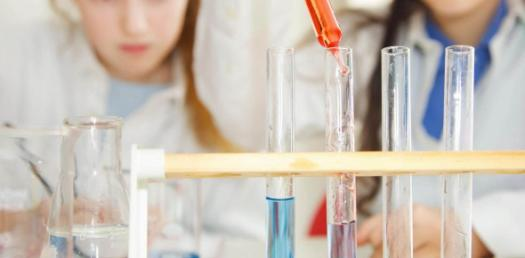# Quiz: Take The Ultimate Chemistry Exam Test!

23 Questions | Total Attempts: 335Settings.

• 1.
Which one of the following is most likely to be an ionic compound?
• A.

CH4

• B.

O2

• C.

CaCl2

• D.

NH3

• 2.
Which of the following compounds has the greatest lattice energy?
• A.

KBr

• B.

KCl

• C.

KI

• D.

KF

• 3.
Based on the tendency of atoms to gain or lose electrons to fulfill the octet rule, which of the following ions has an incorrect charge?
• A.

Na+

• B.

O2-

• C.

Ca2-

• D.

Cl-

• 4.
Which of the following diatomic molecules has the longest bond length?
• A.

N==N

• B.

Br-Br

• C.

F-F

• D.

O==O

• 5.
The measure of an atoms ability to attract electrons towards itself in a chemical bond is known as?
• A.

Electronegativity

• B.

Bond strength

• C.

Electron affinity

• D.

Bond order

• 6.
Which of the following compounds has the greatest bond polarity?
• A.

Cl-Br

• B.

Cl-Cl

• C.

NH3

• D.

H-F

• 7.
Calculate the oxidation number of the nitrogen atom in NH3.
• A.

0

• B.

+1

• C.

-1

• D.

-3

• 8.
Which metal would most likely have the highest melting point?
• A.

Li

• B.

Na

• C.

Ca

• D.

K

• 9.
Which bond type best describes the type of bond that will form between O and H?
• A.

Nonpolar convalent

• B.

Mostly ionic

• C.

Mostly covalent

• D.

Polar covalent

• 10.
Based on your knowledge of bond energy, which of the following compounds would release the most energy during combustion?
• A.

CH4

• B.

C2H6

• C.

C3H8

• D.

C2OH6

• 11.
Use VSEPR theory to predict the geometry of the SF6 molecule.
• A.

T-shaped

• B.

Trigonal bipyramidal

• C.

Tetrahedral

• D.

• 12.
The bond angles found in a molecule with AX5 geometry are?
• A.

90 degrees

• B.

120 degrees

• C.

90 and 120 degrees

• D.

109.5 degrees

• 13.
Which of the following bonds has the most ionic character
• A.

C-H

• B.

H-F

• C.

Na-I

• D.

K-F

• 14.
What is the CN bond order in H3CCN (assume all atoms obey the octet rule)?
• A.

-1

• B.

+1

• C.

0

• D.

+3

• 15.
What is the formal charge on the nitrogen in hydroxylamine, H2NOH?
• A.

-1

• B.

0

• C.

+1

• D.

+5

• 16.
Which of the following has the smallest bond angles?
• A.

CO2

• B.

NH3

• C.

SO3

• D.

XeF4

• 17.
Which molecule has no dipole moment?
• A.

BrF3

• B.

SO2

• C.

XeBr4

• D.

H2O

• 18.
Which of the following molecules is linear?
• A.

NH3

• B.

H2O

• C.

H2S

• D.

CO2

• 19.
Which of the following statements is true of methane, CH4?
• A.

It is a tetrahedral molecule

• B.

It is a polar molecule

• C.

It contains single and double bonds

• D.

It contains 60% nitrogen by weight

• 20.
Using the bond energies, calculate the enthalpy change for the following combustion reaction.
• A.

-92 kJ/mol

• B.

+921 kJ/mol

• C.

-621 kJ/mol

• D.

+621 kJ/mol

• 21.
In the Lewis structure of nitrogen dioxide, NO2, how many pairs of electrons are bonding and how many are non-bonding?
• A.

5 pairs bonding, 4 pairs non-bonding

• B.

3 pairs bonding, 4 pairs non-bonding

• C.

4 pairs bonding, 4 pairs non-bonding

• D.

2 pairs bonding, 2 pairs non-bonding

• 22.
Indicate the molecular geometry and the net dipole moment of H3CF
• A.

Tetrahedral, AX4; net dipole moment arrow pointing to the right

• B.

Pyramidal; net dipole moment arrow point to the left

• C.

Bent; net dipole moment arrow pointing to the right

• D.

Planar tetrahedral; net dipole moment arrow pointing to the right

• 23.
Briefly explain why Co2 is non-polar and OCS is polar.
• A.

CO2; O==C==O no dipole moment, cancels each out; OCS; O==C==S, contains a dipole moment,does not cancel each out

• B.

CO2 O==C==O contains a dipole moment, does not cancel each other out; OCS O==C==) no dipole moment, cancel each out

Related TopicsBack to top